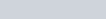# Harmonic motion with inertial reference frames

This post is similar to the post on light clocks here. Simple harmonic motion (SHM) is like the spring below:The equation for describing the period is

where T is the period, m is the mass, and k is the spring constant.

The displacement for each cycle is zero since it returns to its starting point. In a stationary frame, the displacement, x, is a function of the amplitude, A, the angular velocity ω, and the time:The total distance traversed in each cycle is 4A and the total time for each cycle is T, so the average speed for a cycle is 4A/T in a stationary frame.

To determine the average speed relative to an inertial frame moving with velocity v parallel to the SHM, break the cycle into the part moving in the positive direction (I) and the negative direction (II).

(I) the average speed is (4A/T) + v.

(II) the average speed is −((−4A/T) + v) = (4A/T)v.

Then the average speed for each cycle is again 4A/T.

Thus the average speed for each cycle of SHM is constant for all inertial frames of reference.